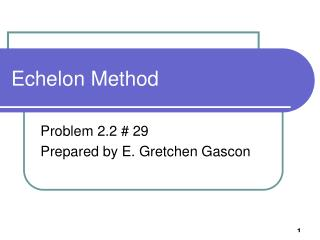DownloadDownload PresentationEchelon Method

# Echelon Method

Télécharger la présentation## Echelon Method

- - - - - - - - - - - - - - - - - - - - - - - - - - - E N D - - - - - - - - - - - - - - - - - - - - - - - - - - -
##### Presentation Transcript

1. Echelon Method Problem 2.2 # 29 Prepared by E. Gretchen Gascon

2. Step 1: The problem Notice that this problem has three unknowns, but only two equations. To arrive at a unique solution, there should be the same number of equations as the number of variables. We will start the problem the same way with the regular echelon method.

3. Step 2: Eliminate the y term Use equation 1 and 2 Reorganize the equations so that the y term is first. You don’t have to but doing so, makes the first step easier. Add the two equations to get a new equation. Remember this equation it will be used later

4. The new system of equations This is the new system of equation. Because there was not a third equation, it was not possible to create a second equation from which the y was eliminated.

5. Step 7: Solve for y and x in terms of z Solve for x in terms of z Assume that z is the parameter. The variable that can be any value. Using the value for x, solve for y in terms of z

6. Comments • Was there anything about this PowerPoint presentation that you would like further explained? • Post comments or questions to the Main Forum.